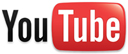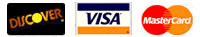CALL US: (814) 654-7509# A unique idea for wall coverings! Let Design-R-Wall help you with wall covering ideas!

Estimating Guide
1. Add the length and width of the room together and double it.
EXAMPLE: 12' + 22' = 34' X 2= 68'

2. Multiply the answer by the ceiling height.
EXAMPLE: 68' X 8' = 544' total square feet of room.

3. Figure the square footage of the area not to be covered, doors and windows.
EXAMPLE: 4.5' X 3.5' = 15.75 X 2 = 31.5 window sq. ft.;
82 + 41 = 123 sq. ft. of door; 31.5 + 123 = 154.5 sq. ft. not covered.

4. Subtract the above number from the total square feet.
EXAMPLE: 544' - 154.5' = 389.5 sq. ft.

5. Divide the total square feet by 40.
EXAMPLE: 389.5 sq. ft. / 40 = 9.73 or 10 bags.